# 全文概要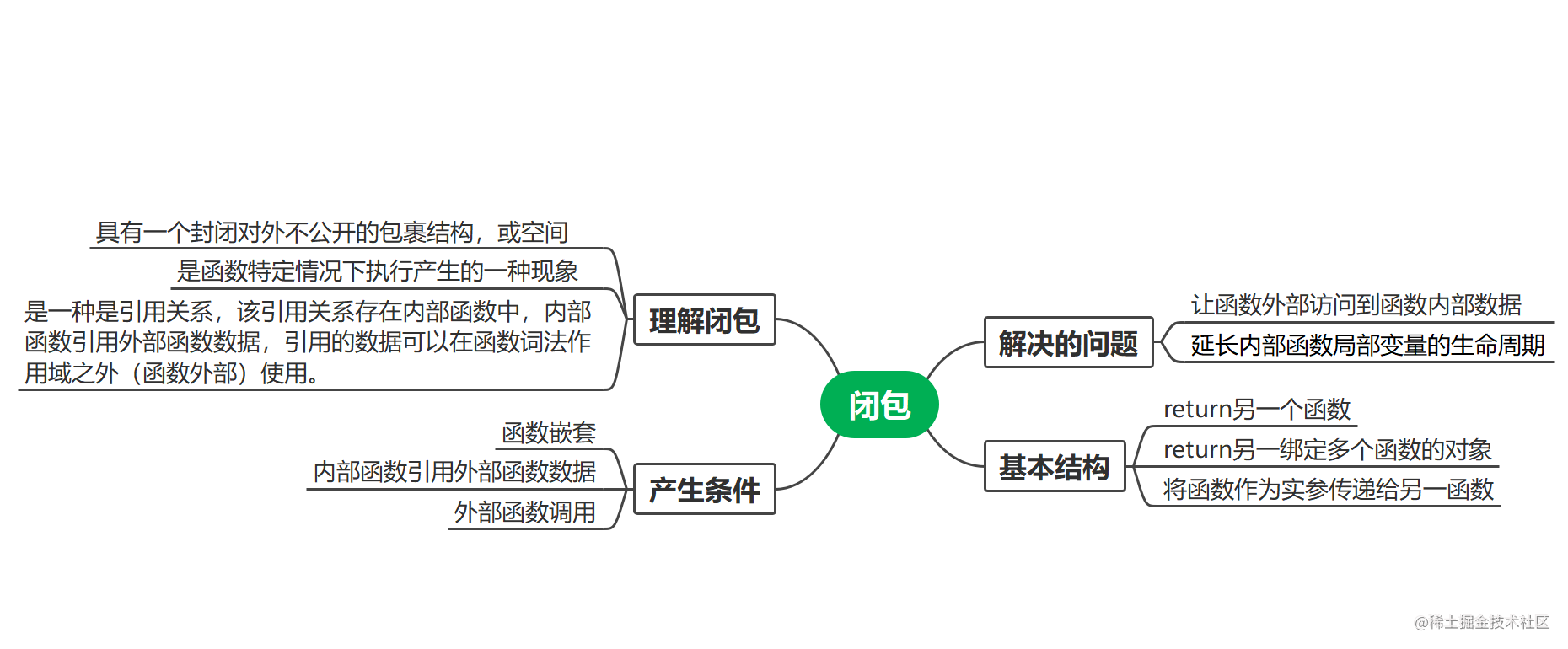# 2 解决的问题

``````  function f1(){
var num = 10;
return num;
}
var res = f1();
console.log(res); // 10

``````function f1() {
var num = Math.random();
return num;
}
var res = f1();
var res2 = f1();
console.log(res + '\n' + res2);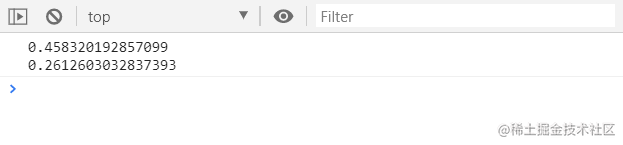``````function f1(){
var num = Math.random();

function f2(){
return num
}

return f2
}

var f = f1();
var res1 = f();
var res2 = f();
console.log(res + '\n' + res2);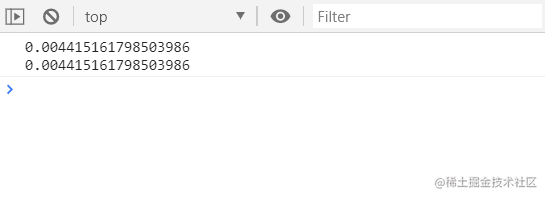这就产生了闭包，我们试着分析一下这段代码：

• 全局`f1`函数在0级作用域链上，`f1`函数是一个一级链，`f1`函数中有一个变量`num`，还有一个函数体`f2`

• `f2`是二级链，通过return将`f2`当做一个值返回给`f1`函数。

• `f1`函数执行后，将`f2`的引用赋值给`f`，执行`f`函数，输出`num`变量。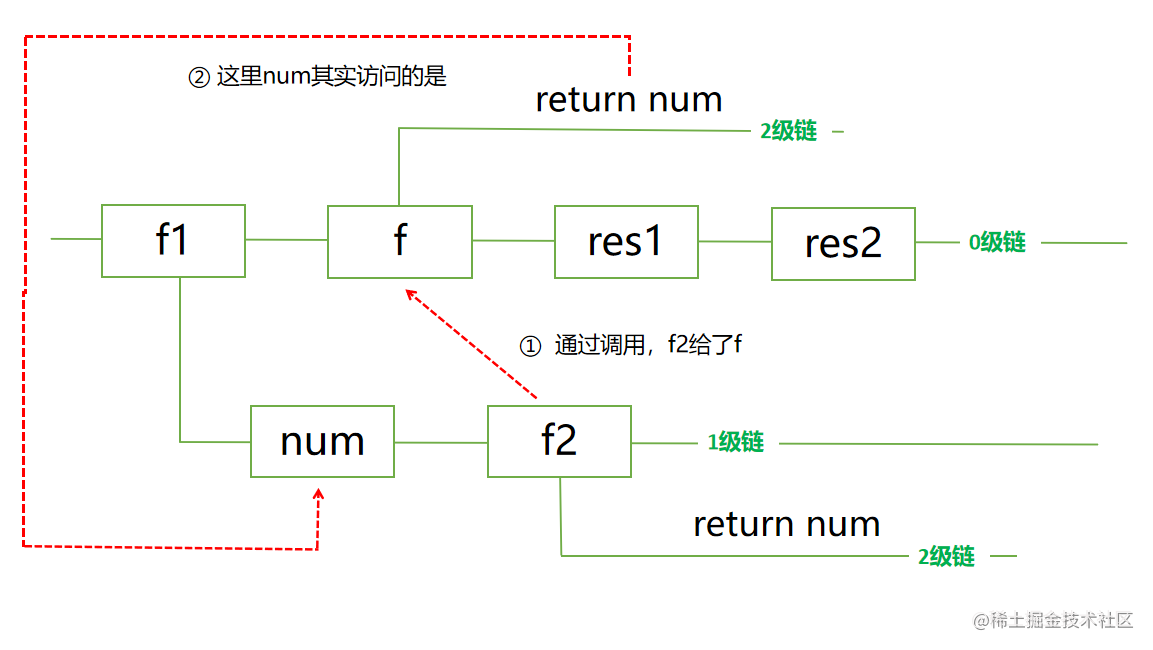# 3 闭包本质

``````  function f1() {
var num = Math.random();
function f2() {
return num;
}

return f2;
}

f1()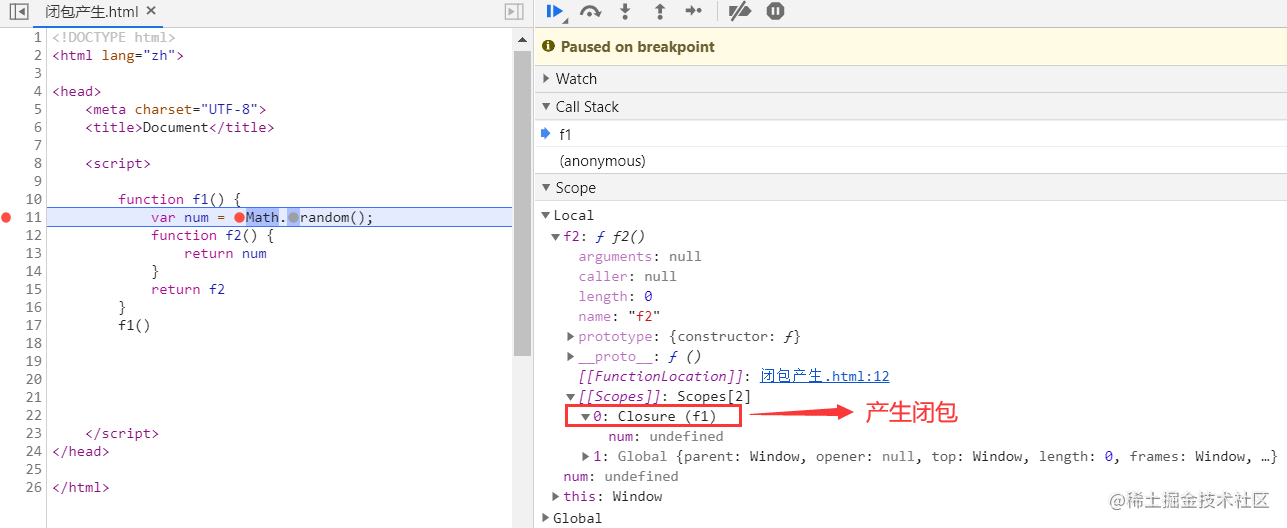# 4 基本结构

## 4.1 return另一函数

``````function f1(){
var a = 0
function f2(){
a++
console.log(a)
}
return f2
}
var f = f1();
f(); // 1
f(); // 2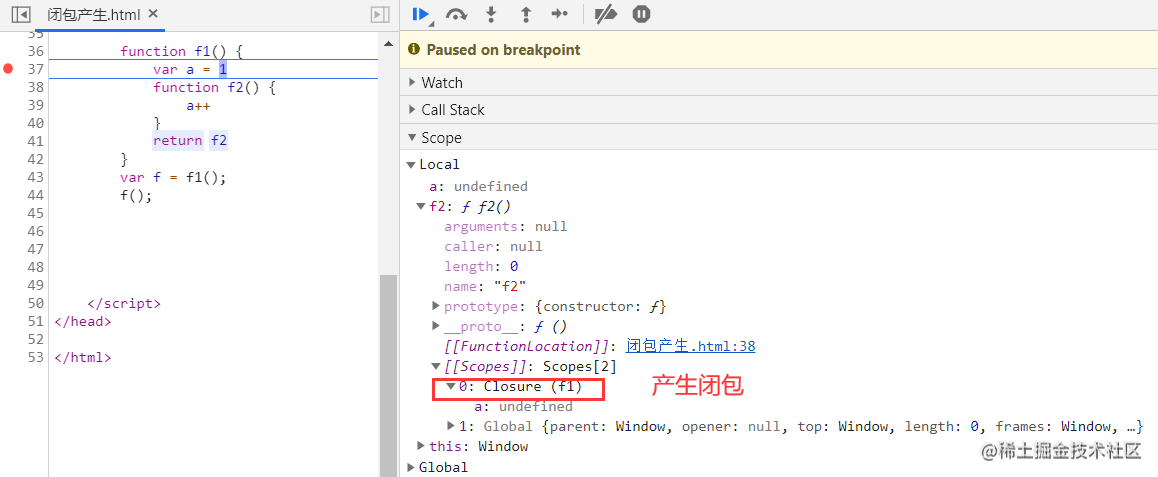## 4.2 return绑定多个函数的对象

eg : 如何获得超过一个数据？

``````function f1(){
var num1 = Math.random();
var num2 = Math.random();

return{
num1:function(){
return num1;
},
num2:function(){
return num2
}
}
}

f1()
// {num1: ƒ, num2: ƒ}

f = f1()
f.num1()
f.num2()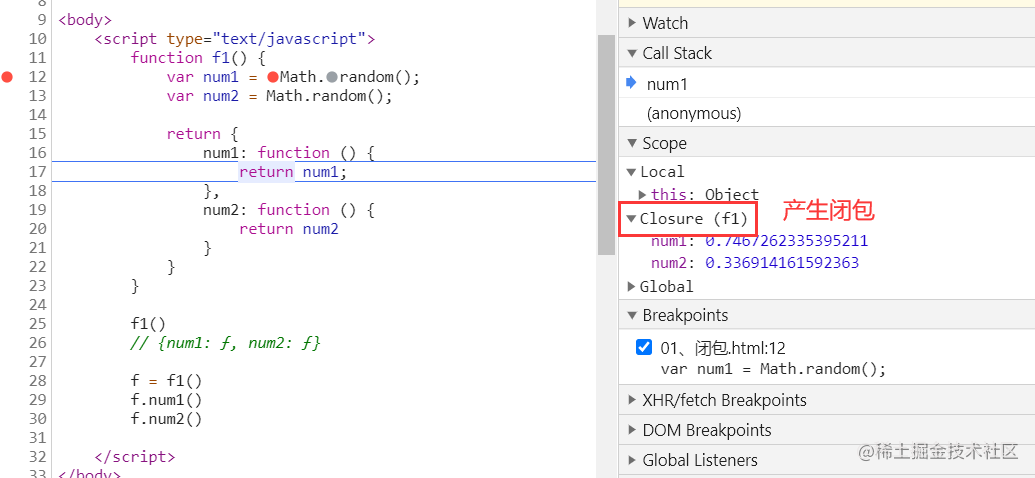eg: 如何读取一个数据和修改一个数据？

``````function f1(){
var num =Math.random();
return {
get_num:function(){
return num;
},
set_num:function(value){
// 此时num访问的是f1函数中的num
num = value;
}
}
}

var f = f1();

// 读取函数中的值
var num = f.get_num();
console.log(num);
// 0.3919299622715364

// 设置函数中的值
f.get_num(123);
num = f.get_num();
console.log(num);
//123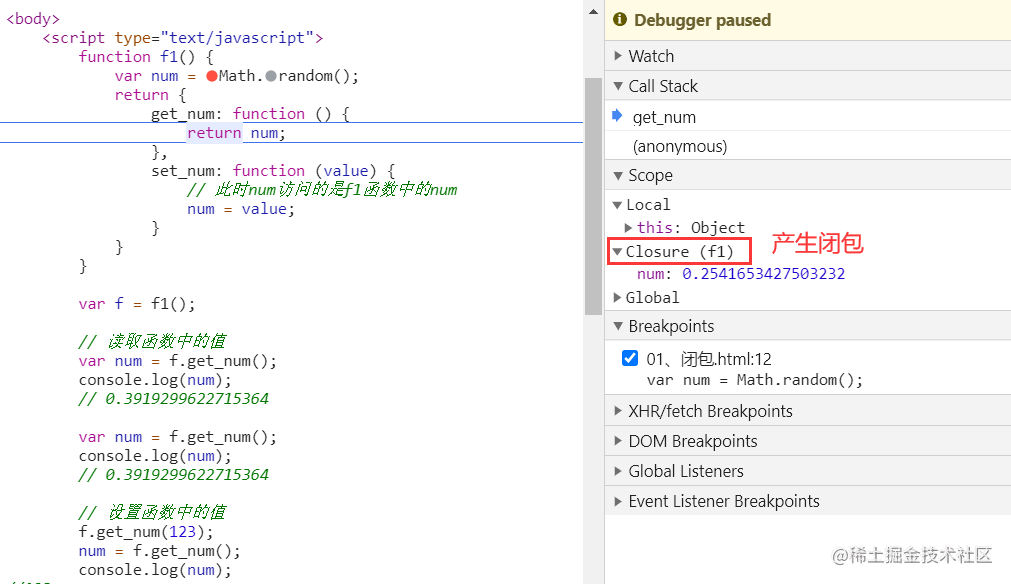## 4.3 将函数实参传递给另一函数

``````function delay(msg){
setTimeout(function(){
console.log(msg)
},2000)
}
delay('开启计时器')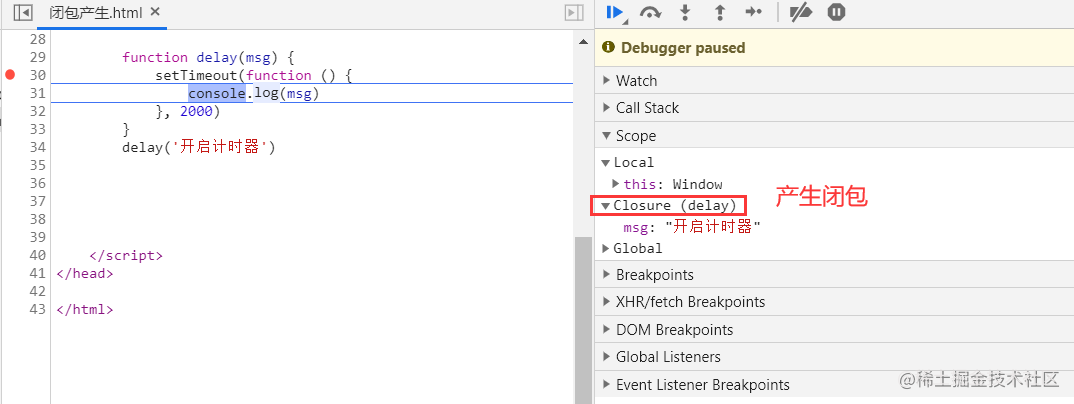# 5 总结

• 闭包：具有对外封闭不公开的包裹结构或空间，函数可以构成闭包。
• 闭包产生三要素：函数嵌套、内部函数引用外部函数数据、外部函数调用。
• 所谓闭包，是一种引用关系，该引用关系存在内部函数中，内部函数引用外部函数数据，引用的数据可以在函数词法作用域（函数外部）之外使用。
• 解决的问题：间接访问函数中的数据、延长内部函数局部变量的生命周期。
• 闭包本质：内部函数里的一个对象，这个对象非Js对象（有属性有方法的对象），这个对象是函数在运行时，本该释放的活动对象，这个活动对象里包含着我们引用的变量
• 基本结构：rerurn另一函数、return绑定多个函数的对象、将函数实参传递给另一函数。